# DAY Excel FunctionArticle byExcelMojo Team## What Is DAY Function In Excel?

The DAY function in Excel returns the sequence of a day in a month in numbers. The function calculates the current day in a month, as there are 31 Days maximum on any day of the year. Still, if the entered number is greater than 31 or above, due to some data formatting, the Day function automatically converts that day sequence as per the day falling in that sequence month since we have numbers between 1 to 365.

For example, consider the below table with a date in cell A2. Now, we need to use the below steps to obtain the result using DAY Excel Function.

First, select the cell to display the result. In this example, we have selected cell B2. Next, enter the DAY Function in cell B2. So, the complete formula is =DAY(A2).

Then, press Enter key. Clearly, the output is returned as 1 in cell B2. Likewise, we can fetch the days using DAY Excel Function.

###### Key Takeaways
• The DAY Excel Function is used to fetch the days in a given data.
• The DAY Excel formula is =DAY(serial_number) and it denotes the date of the day which we are trying to find.
• The “#NUM!” error occurs while using DAY Excel function when the supplied argument is a numeric value but is not recognized as a valid date.
• The “#VALUE!” error occurs when the supplied argument is a text value and cannot be considered a valid date.
• The DAY Excel formula helps in modeling many business models.

### DAY() Excel Formula

The syntax of the DAY Excel Formula is

where,

• serial_number: It is the mandatory argument and it denotes the date of the day which we are trying to find. The dates to the DAY function can be entered as serial numbers, date values returned from other excel functions, and references to cells containing dates.

### How To Use DAY Excel Function?

We can insert DAY excel function using the below steps:

#### 1. Access From The Excel Ribbon

• First, choose the empty cell which will contain the result.
• Next, go to the Formulas tab.
• Then, select the Date & Time option from the menu.
• Select DAY from the drop-down menu.
• A window called Function Arguments appears.
• As the number of arguments, enter the value in the serial_number.
• Select OK.

#### 2. Enter The Worksheet Manually

1. To begin with, select an empty cell for the output.
2. Next, type =DAY() in the selected cell. Alternatively, type =D and double-click the DAY function from the list of suggestions shown by Excel.
3. Then, press Enter key.

The below table shows date in default format in column A. We need to use the below steps to obtain the result using DAY function.

The steps to fetch the day with DAY Excel Function are as follows:

1. To begin with, select the cell where we will enter the formula and calculate the result. The selected cell, in this case, is cell B2.

2. Next, we will enter the DAY excel formula in cell B2.

3. Then, enter A2 as the serial number value.

4. So, the complete formula is =DAY(A2)in cell B2.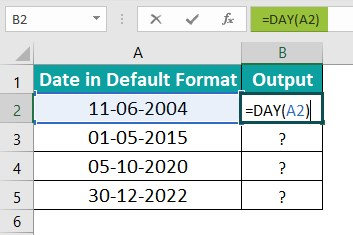5. Next, press Enter key.

We can clearly see that the function has returned the value as 11 in cell B2.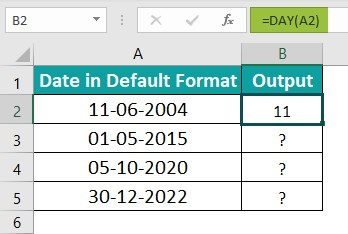6. Now, we can either apply the function manually or use the AutoFill option.

Let us use the AutoFill option and drag the cursor to cell B5 as shown in the following image.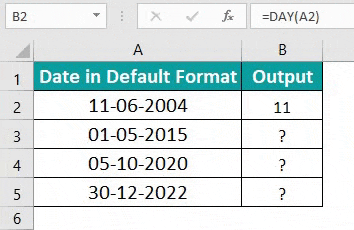### Examples

#### Example #1

The below table shows the date values in full format in column A. Now, we need to use the below steps to calculate the day using DAY Excel Function.

The steps to fetch the value with DAY function are as follows:

Step 1: To begin with, select the cell where we will enter the formula and calculate the result. The selected cell, in this case, is cell B2.

Step 2: Next, we will enter the DAY Excel formula in cell B2.

Step 3: Then, enter A2 as the serial number value.

Step 4: So, the complete formula is =DAY(A2).

Step 5: Finally, press Enter key.

Clearly, the output is returned as 11 in cell B2.

Step 6: Now, let us use the AutoFill option and drag the cursor to cell B5 as shown in the following image.

Similarly, we can use DAY Function to fetch the days.

#### Example #2

The following table shows the date values. Now, we need to calculate the date after adding the given number of years using DAY, MONTH & DATE Excel functions.

In the table,

• Column A shows the date values.
• Column B displays the year.

The steps to find the output with DAY Excel Function are as follows:

Step 1: First, select the cell where we will enter the formula and calculate the result. The selected cell, in this case, is cell C2.

Step 2: Next, we will enter the DAY with DATE Excel function and MONTH formulas in cell C2.

Step 3: Then, enter A2 as the serial number value.

Step 4: So, the complete formula is =DATE(YEAR(A2)+B2,MONTH(A2),DAY(A2))in cell C2.

Step 5: Next, press the Enter key.

Clearly, we can see that the function has obtained the result in cell C2 as ‘11 June 2014’ as shown in the image below.

Step 6: Now, let us use the AutoFill option and drag the cursor to cell C5 as shown in the following image.

Likewise, we can obtain the desired results using DAY Function.

#### Example #3

The following table shows dates in various formats in column A. Now, we need to calculate the first day of the month using DAY Excel function.

The steps to fetch values with DAY Excel function are as follows:

Step 1: To begin with, select the cell where we will enter the formula and calculate the result. The selected cell, in this case, is cell B2.

Step 2: Next, we will enter the DAY Excel formula in cell B2.

Step 3: Enter A2 as the serial number value.

Step 4: So, the complete formula is =A2-DAY(A2)+1 in cell B2.

Step 5: Now, press the Enter key.

Clearly, we can see that the function has returned the result in cell B2 as ‘01 June 2004’ as shown in the image below.

Step 6: Now, we can either apply the function manually or use the AutoFill option.

Now, let us use the AutoFill option and drag the cursor to cell B5 as shown in the following image.

Also, the formula used in each cell is shown in column C.

Thus, we can obtain the exact days using DAY Excel Function.

### Important Things To Note

• The DAY function in Excel contains various applications within the spreadsheet.
• The date inserted in the DAY formula must be a valid Excel date in the serial number format.
• Microsoft Excel can only read dates after 1/1/1900.
• The DAY function gives the result that looks like a date ‘01/01/1900’ instead of an integer.
• Some of the common applications of the DAY function in spreadsheets are:
• To get a series of dates by year, month
• To add years to date or specific date
• To get a day from the date or first day of the month

1. What is the use of DAY Excel function?

The DAY Excel function means fetching the exact day from the given date. The argument for the DAY function is ‘serial_number.’ This mandatory argument refers to the date of the day which we are trying to find. The DAY Function is categorized under Excel Date & Time Function.

2. How to insert DAY Excel function?

We can insert DAY function using the below steps:

#1 – Select an empty cell for the output.
#2 – Type =DAY() in the selected cell.
Alternatively, type =D and double-click the DAY function from the list of suggestions shown by Excel.
#3 – Press Enter key.

For example, the following table shows the values in column A. We need to use the below steps to obtain the result using DAY Excel Function.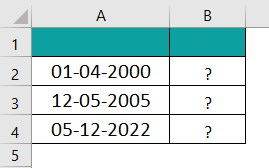The steps to find the values using DAY Excel function are as follows:

Step 1: To begin with, select the cell where we will enter the formula and calculate the result. The selected cell, in this case, is cell B2.
Step 2: Next, we will enter the DAY Excel formula in cell B2.
Step 3: Then, enter A2 as the serial number value.
Step 4: So, the complete formula is =DAY(A2)in cell B2.
Step 5: Now, press the Enter key.
We can see that the function has returned the result in cell B2 as ‘1’ in the image below.
Step 6: Finally, press Enter and drag the cursor to cell B4, as shown in the following image.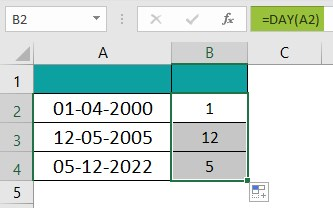3. Where is the DAY function in Excel?

We can activate DAY Excel function using the following steps:

· To begin with, choose the empty cell which will contain the result.
· Next, go to the Formulas tab.
· Then, select the Date & Time group.
· Select DAY from the drop-down menu.
· The Function Arguments window appears.
· Based on the number of arguments, enter the value in the serial_number argument.
· Select OK.